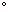Name _____________________________Date ___________________
Isosceles Triangles
Complete.

 1 The base angle of an isosceles triangle is 57. What is the measure of the vertex angle?
 2 The vertex angle of an isosceles triangle is 34. What is the measure of the base angle?
 3 The degree measure of the vertex angle is (4 + 12x). The degree measure for each base angle is (53 + x). What is the value of vertex angle?
 4 The ratio of the measure of a base angle in an isoceles triangle to the measure of the vertex angle is 14:8. Find the measure of each angle.
 5 The degree measure of the vertex angle is (7x - 9). The degree measure for each base angle is (2x + 56). What is the value of base angle?
 6 The degree measure of the vertex angle is (6x - 38). The degree measure for each base angle is (4x - 38). What is the value of x?
 7 The measure of the exterior angle at the base an isosceles triangle is 143. What is the measure of the vertex angle?
 8 The degree measure of the vertex angle is (x + 96). The degree measure for each base angle is (33 + x). What is the value of the measure of an exterior angle at the base of the triangle?
 9 The degree measure of the vertex angle is (17x - 2). The degree measure for each base angle is (8x - 8). What is the value of the measure of an exterior angle at the base of the triangle?
 10 The ratio of the measure of a base angle in an isoceles triangle to the measure of the vertex angle is 1:3. Find the measure of each angle.